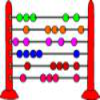#### You may also like### Which Scripts?

There are six numbers written in five different scripts. Can you sort out which is which?### A Romantic Riddle

Read this riddle and see if you can work out how the trees must be planted.### Fibonacci's Three Wishes 1

First of two articles about Fibonacci, written for students.

# Three Neighbours

##### Age 7 to 14Challenge Level

Three Neighbours printable sheet

5, 6 and 7 are three consecutive numbers. They add up to 18.

14, 15 and 16 are also three consecutive numbers. They add up to 45.

Take other sets of three consecutive numbers and find their total.

What do you notice?
Do the totals have anything in common?

How can you be sure that what you have noticed will always be true?

Mathematicians aren't usually satisfied with a few examples to convince themselves that something is always true.
Have you been able to provide an argument that would convince mathematicians?

Liz noticed that all the totals are a multiple of 3. She found it useful to draw a picture:How did this help to convince Liz that three consecutive numbers always add up to a multiple of 3?

Charlie also noticed that all the totals were a multiple of 3. He thought about sets of numbers in a systematic way:

Charlie started with the three consecutive numbers 1, 2, 3. They add up to 6.

He added 1 to each, which gave him the next three consecutive numbers 2, 3, 4. They add up to 9.

He added 1 to each again, and ended up with 3, 4, 5. They add up to 12.

How did this help to convince Charlie that three consecutive numbers always add up to a multiple of 3?

If you have met algebra before, then you might like to look at Claire's method:

Claire decided to call the first number $n$.

The next two numbers are then equal to $n+1$ and $n+2$.

Claire added the three numbers to get $n+ n+1 + n+2 = 3n + 3$.

How did this help to convince Claire that three consecutive numbers always add up to a multiple of 3?

What happens when you add five consecutive numbers? Seven consecutive numbers? ...